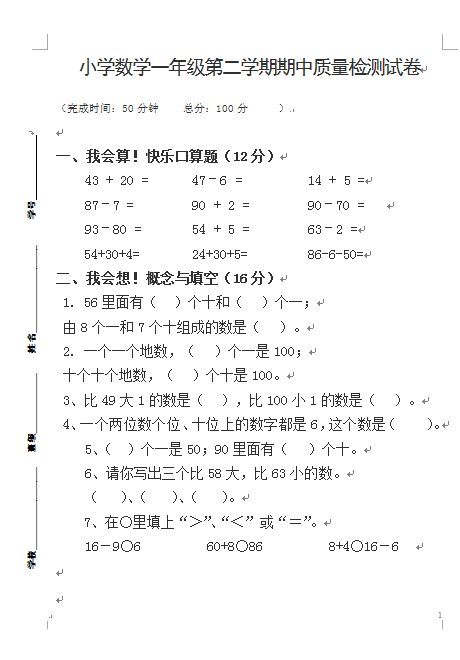（完成时间：50分钟     总分：100分      ）

一、我会算！快乐口算题（12分）

43 + 20 =      47–6 =         14 + 5 =

87–7 =        90 + 2 =        90–70 =

93–80 =       54 + 5 =        63–2 =

54+30+4=       24+30+5=        86-6-50=

【DOC文档4页】数学小学一年级第二学期期中质量检测试卷A4电子版资料_可直接打印_会员免费下载##### 评论信息